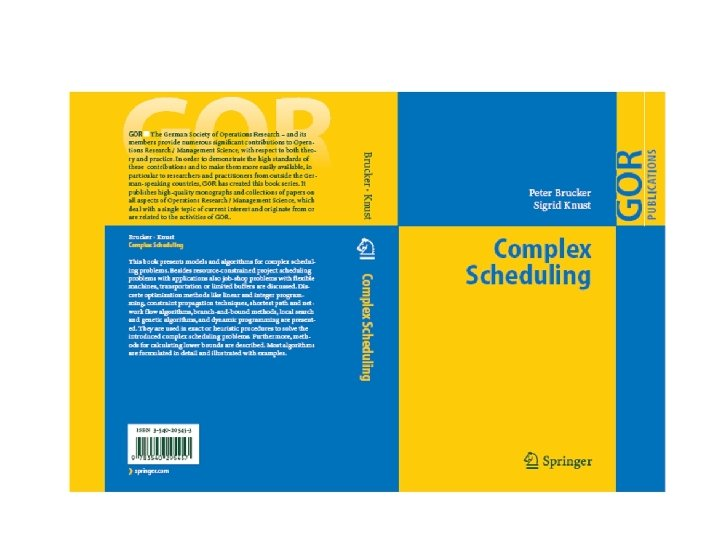Скачать презентацию On the Complexity of Scheduling Peter Brucker University

9eda35d45dc6ab374019771bb1fed241.ppt

• Количество слайдов: 61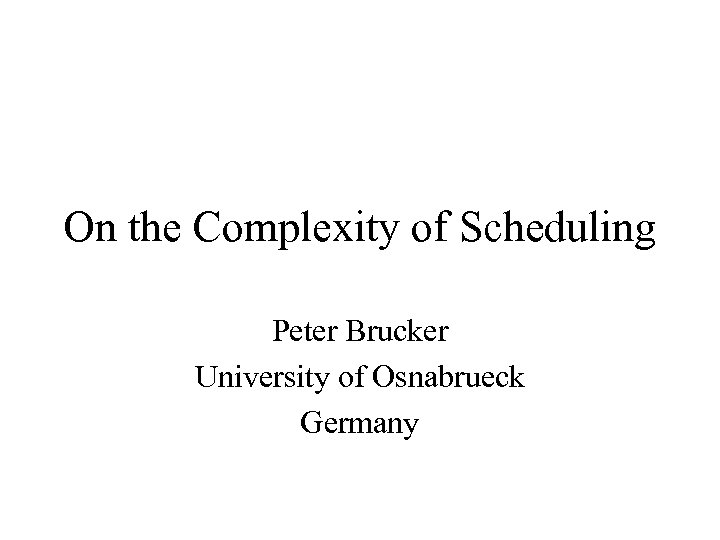On the Complexity of Scheduling Peter Brucker University of Osnabrueck Germany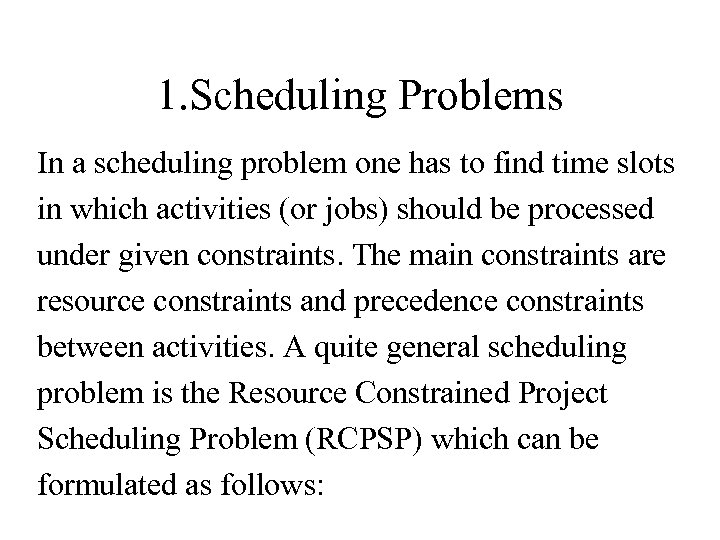1. Scheduling Problems In a scheduling problem one has to find time slots in which activities (or jobs) should be processed under given constraints. The main constraints are resource constraints and precedence constraints between activities. A quite general scheduling problem is the Resource Constrained Project Scheduling Problem (RCPSP) which can be formulated as follows: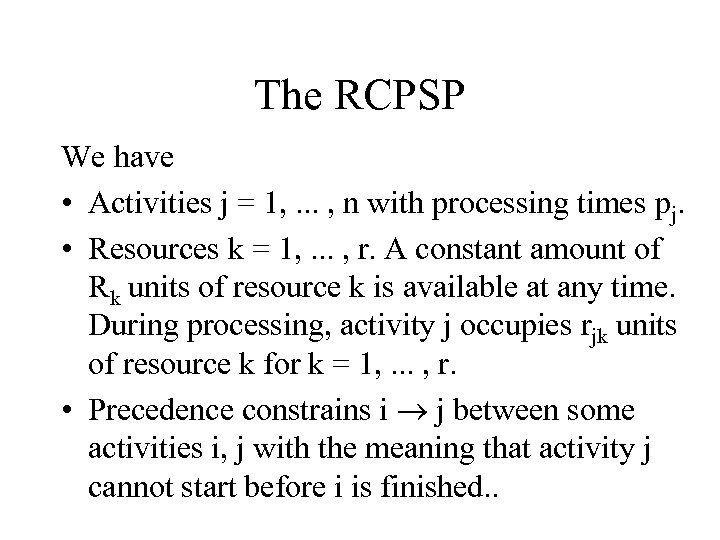The RCPSP We have • Activities j = 1, . . . , n with processing times pj. • Resources k = 1, . . . , r. A constant amount of Rk units of resource k is available at any time. During processing, activity j occupies rjk units of resource k for k = 1, . . . , r. • Precedence constrains i j between some activities i, j with the meaning that activity j cannot start before i is finished. .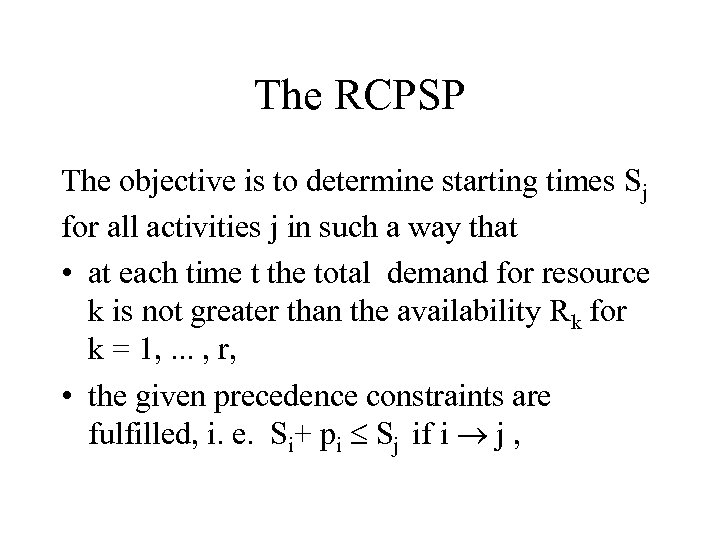The RCPSP The objective is to determine starting times Sj for all activities j in such a way that • at each time t the total demand for resource k is not greater than the availability Rk for k = 1, . . . , r, • the given precedence constraints are fulfilled, i. e. Si+ pi Sj if i j ,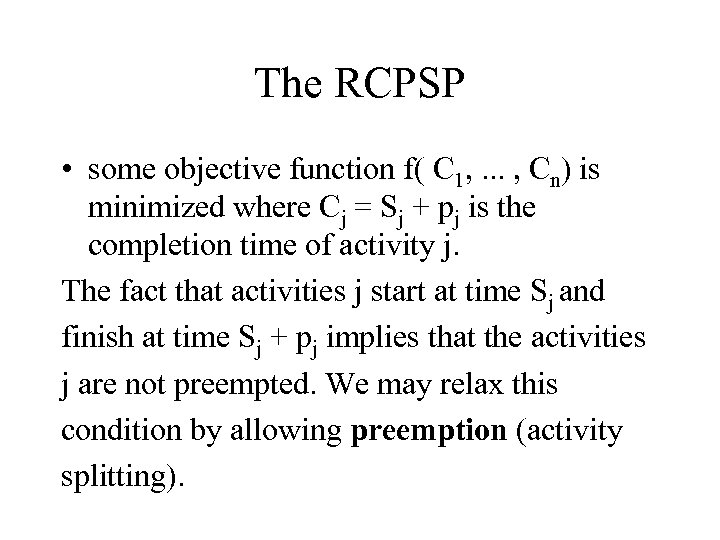The RCPSP • some objective function f( C 1, . . . , Cn) is minimized where Cj = Sj + pj is the completion time of activity j. The fact that activities j start at time Sj and finish at time Sj + pj implies that the activities j are not preempted. We may relax this condition by allowing preemption (activity splitting).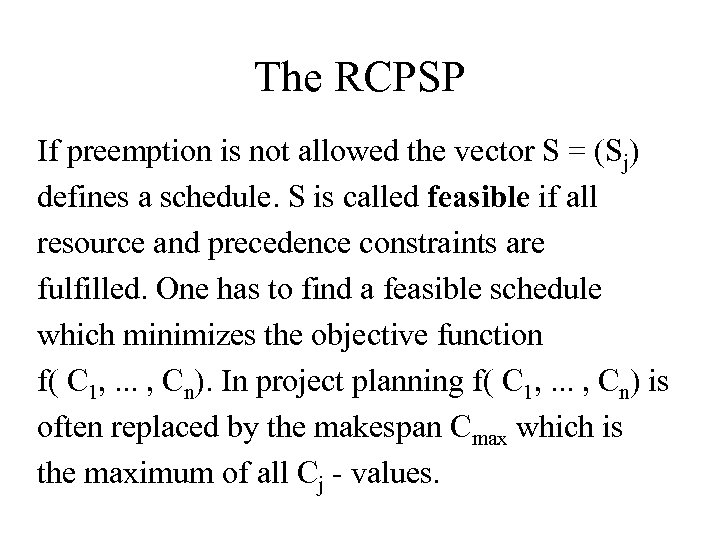The RCPSP If preemption is not allowed the vector S = (Sj) defines a schedule. S is called feasible if all resource and precedence constraints are fulfilled. One has to find a feasible schedule which minimizes the objective function f( C 1, . . . , Cn). In project planning f( C 1, . . . , Cn) is often replaced by the makespan Cmax which is the maximum of all Cj - values.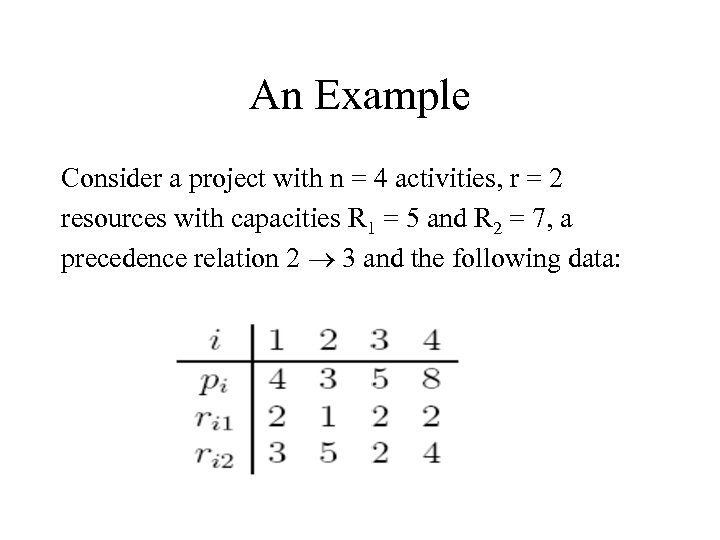An Example Consider a project with n = 4 activities, r = 2 resources with capacities R 1 = 5 and R 2 = 7, a precedence relation 2 3 and the following data: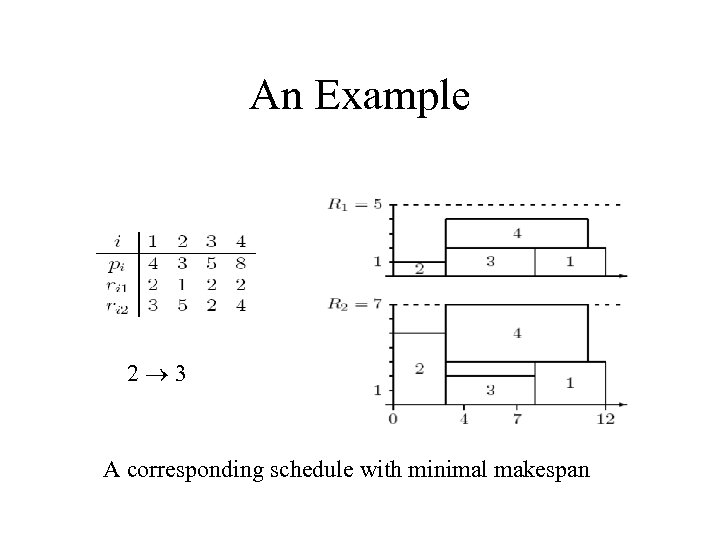An Example 2 3 A corresponding schedule with minimal makespan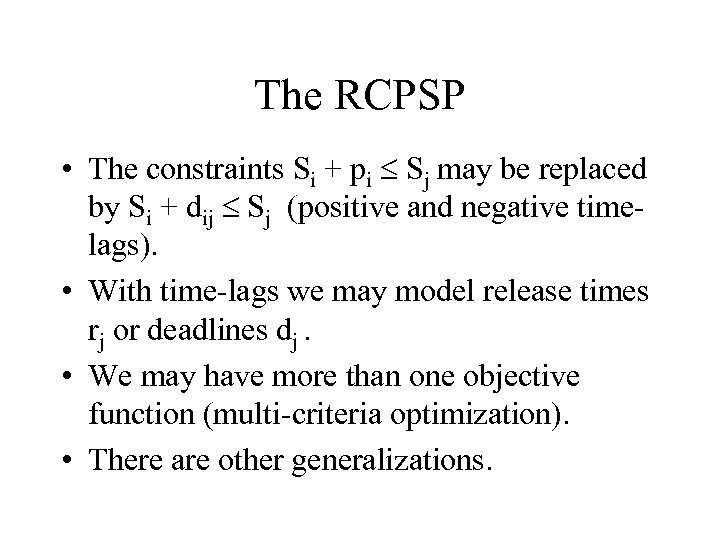The RCPSP • The constraints Si + pi Sj may be replaced by Si + dij Sj (positive and negative timelags). • With time-lags we may model release times rj or deadlines dj. • We may have more than one objective function (multi-criteria optimization). • There are other generalizations.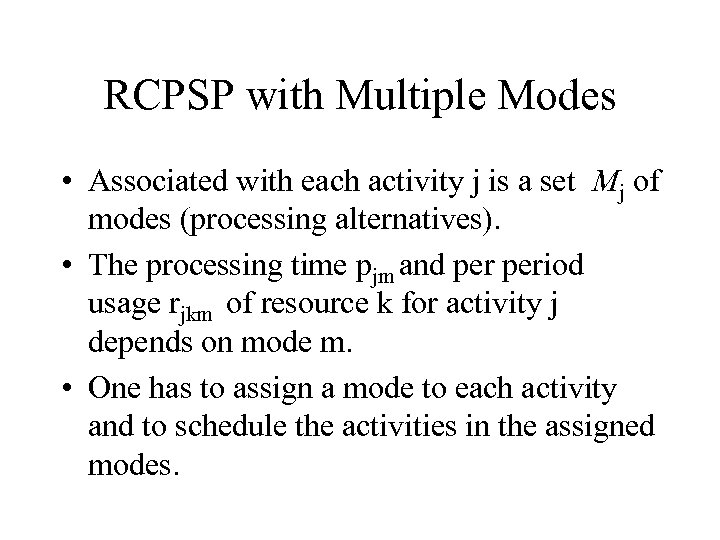RCPSP with Multiple Modes • Associated with each activity j is a set Mj of modes (processing alternatives). • The processing time pjm and period usage rjkm of resource k for activity j depends on mode m. • One has to assign a mode to each activity and to schedule the activities in the assigned modes.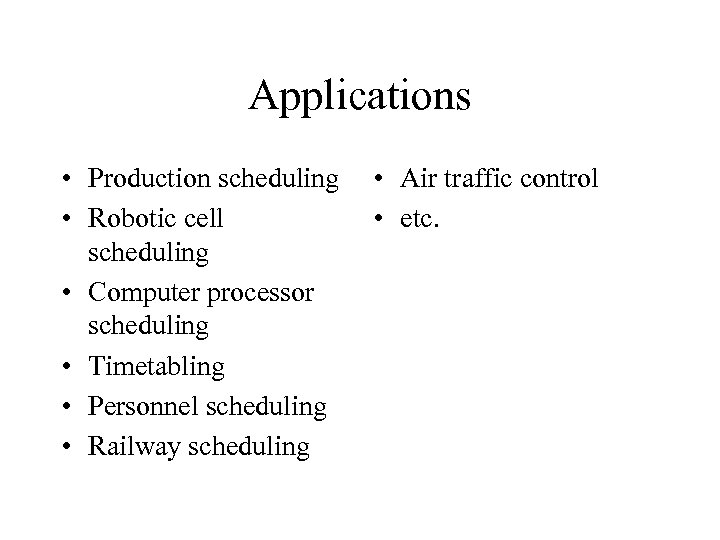Applications • Production scheduling • Robotic cell scheduling • Computer processor scheduling • Timetabling • Personnel scheduling • Railway scheduling • Air traffic control • etc.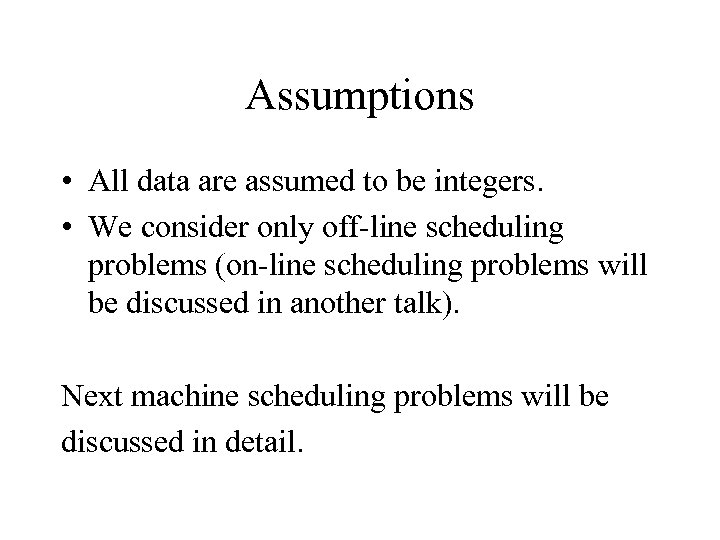Assumptions • All data are assumed to be integers. • We consider only off-line scheduling problems (on-line scheduling problems will be discussed in another talk). Next machine scheduling problems will be discussed in detail.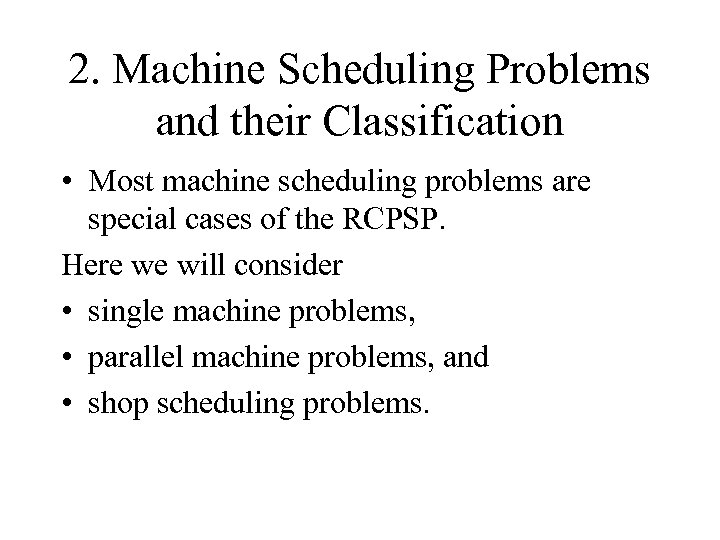2. Machine Scheduling Problems and their Classification • Most machine scheduling problems are special cases of the RCPSP. Here we will consider • single machine problems, • parallel machine problems, and • shop scheduling problems.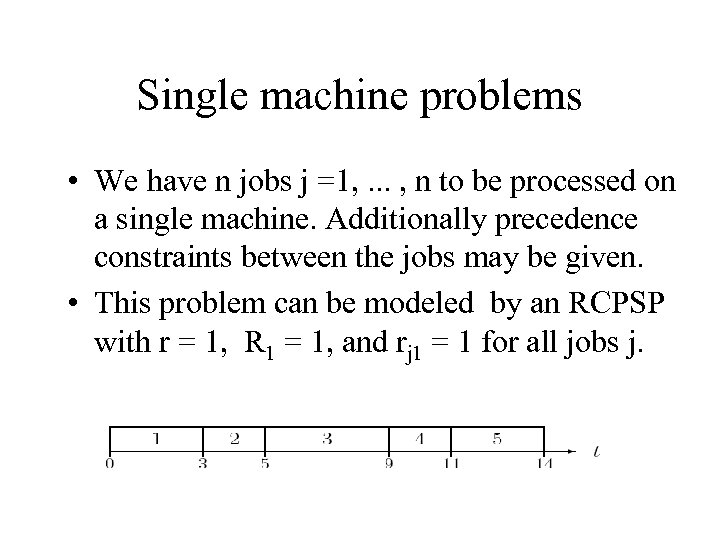Single machine problems • We have n jobs j =1, . . . , n to be processed on a single machine. Additionally precedence constraints between the jobs may be given. • This problem can be modeled by an RCPSP with r = 1, R 1 = 1, and rj 1 = 1 for all jobs j.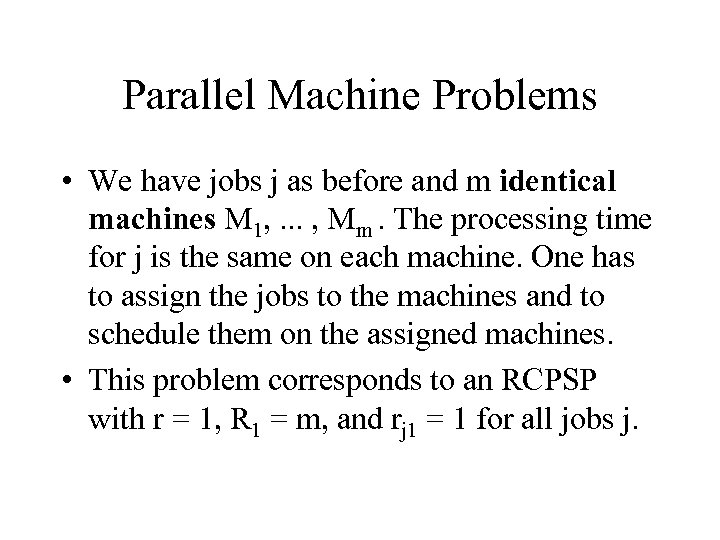Parallel Machine Problems • We have jobs j as before and m identical machines M 1, . . . , Mm. The processing time for j is the same on each machine. One has to assign the jobs to the machines and to schedule them on the assigned machines. • This problem corresponds to an RCPSP with r = 1, R 1 = m, and rj 1 = 1 for all jobs j.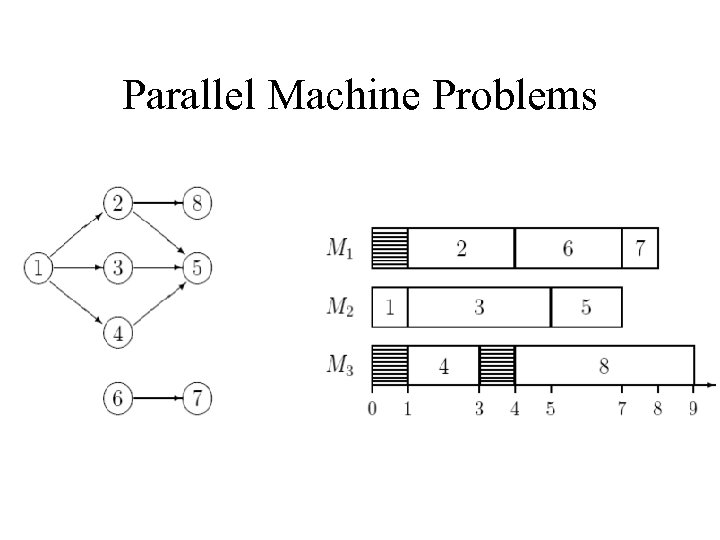Parallel Machine Problems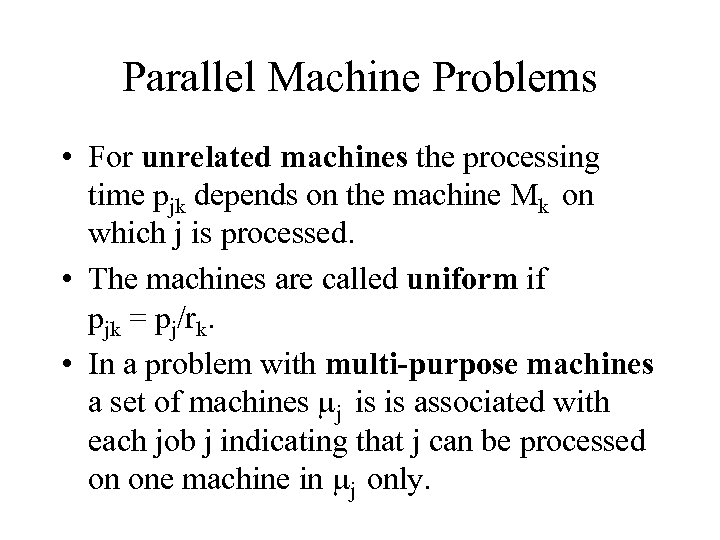Parallel Machine Problems • For unrelated machines the processing time pjk depends on the machine Mk on which j is processed. • The machines are called uniform if pjk = pj/rk. • In a problem with multi-purpose machines a set of machines mj is is associated with each job j indicating that j can be processed on one machine in mj only.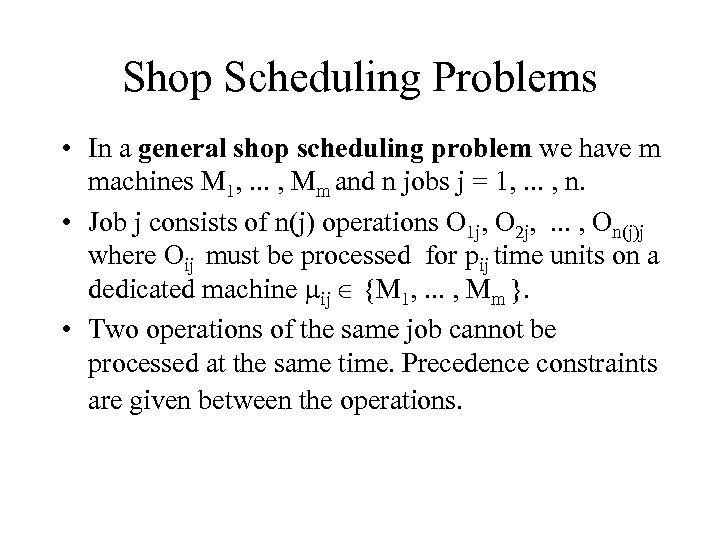Shop Scheduling Problems • In a general shop scheduling problem we have m machines M 1, . . . , Mm and n jobs j = 1, . . . , n. • Job j consists of n(j) operations O 1 j, O 2 j, . . . , On(j)j where Oij must be processed for pij time units on a dedicated machine mij {M 1, . . . , Mm }. • Two operations of the same job cannot be processed at the same time. Precedence constraints are given between the operations.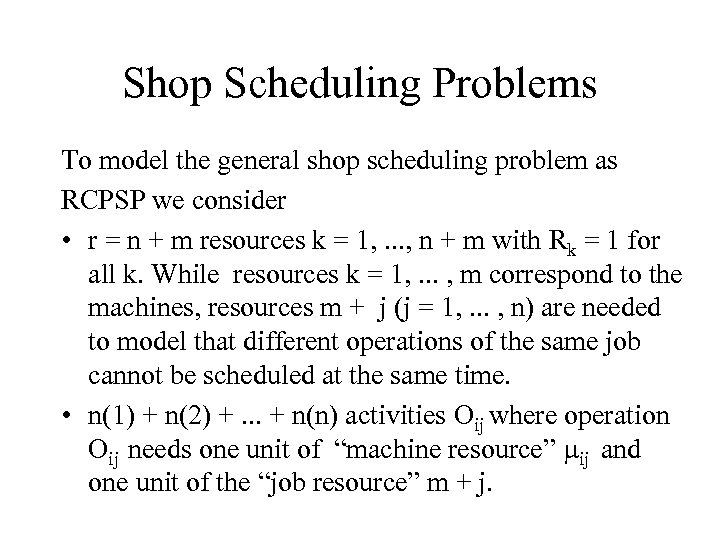Shop Scheduling Problems To model the general shop scheduling problem as RCPSP we consider • r = n + m resources k = 1, . . . , n + m with Rk = 1 for all k. While resources k = 1, . . . , m correspond to the machines, resources m + j (j = 1, . . . , n) are needed to model that different operations of the same job cannot be scheduled at the same time. • n(1) + n(2) +. . . + n(n) activities Oij where operation Oij needs one unit of “machine resource” mij and one unit of the “job resource” m + j.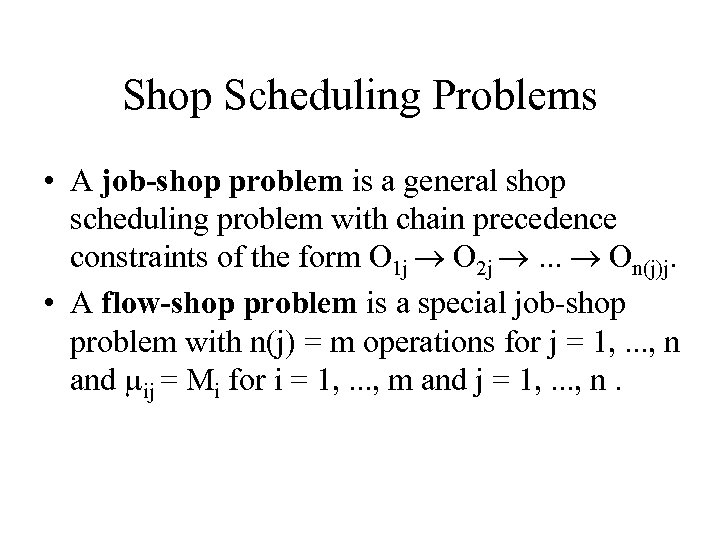Shop Scheduling Problems • A job-shop problem is a general shop scheduling problem with chain precedence constraints of the form O 1 j O 2 j . . . On(j)j. • A flow-shop problem is a special job-shop problem with n(j) = m operations for j = 1, . . . , n and mij = Mi for i = 1, . . . , m and j = 1, . . . , n.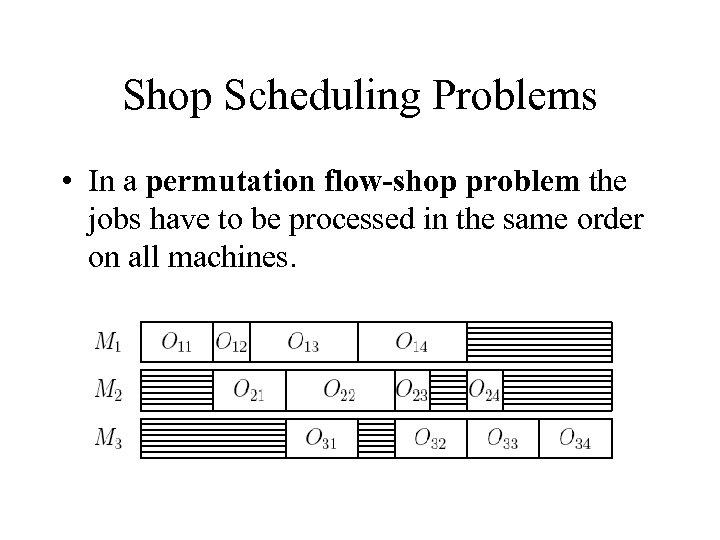Shop Scheduling Problems • In a permutation flow-shop problem the jobs have to be processed in the same order on all machines.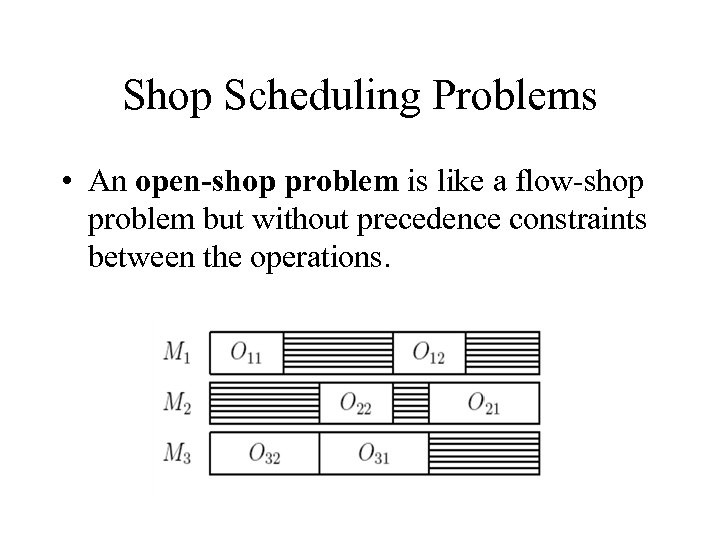Shop Scheduling Problems • An open-shop problem is like a flow-shop problem but without precedence constraints between the operations.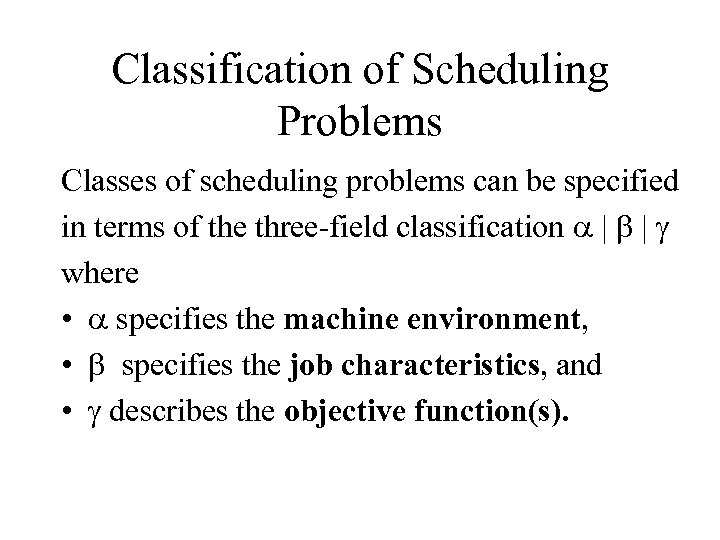Classification of Scheduling Problems Classes of scheduling problems can be specified in terms of the three-field classification a | b | g where • a specifies the machine environment, • b specifies the job characteristics, and • g describes the objective function(s).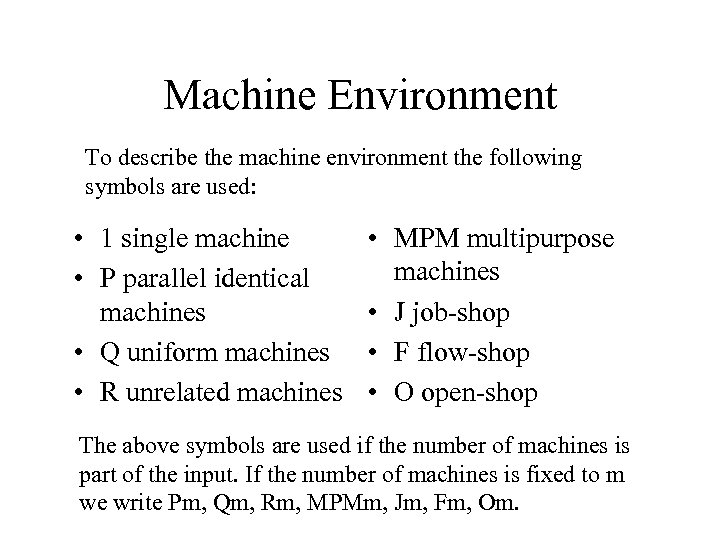Machine Environment To describe the machine environment the following symbols are used: • 1 single machine • P parallel identical machines • Q uniform machines • R unrelated machines • MPM multipurpose machines • J job-shop • F flow-shop • O open-shop The above symbols are used if the number of machines is part of the input. If the number of machines is fixed to m we write Pm, Qm, Rm, MPMm, Jm, Fm, Om.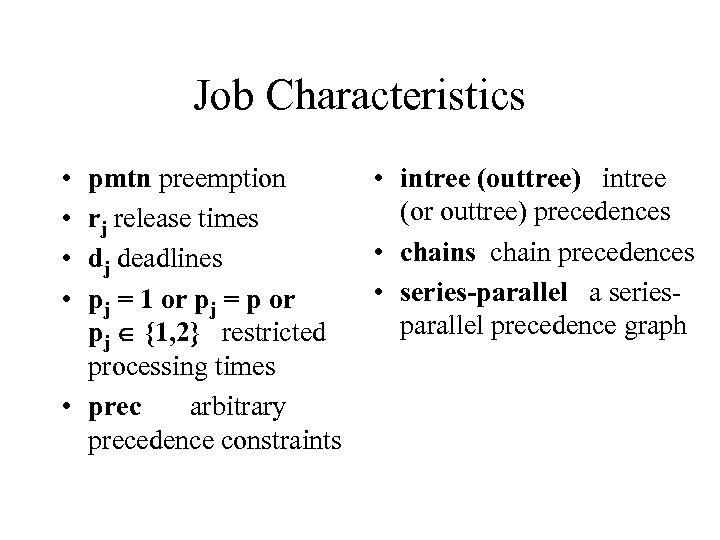Job Characteristics • • pmtn preemption rj release times dj deadlines pj = 1 or pj = p or pj {1, 2} restricted processing times • prec arbitrary precedence constraints • intree (outtree) intree (or outtree) precedences • chains chain precedences • series-parallel a seriesparallel precedence graph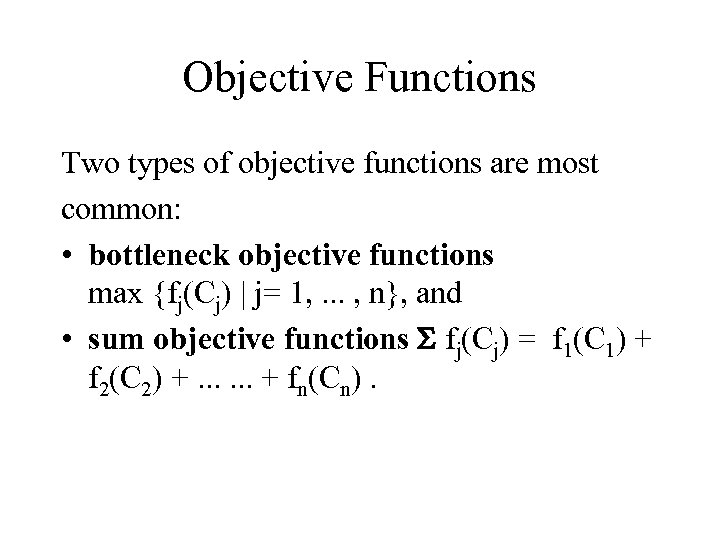Objective Functions Two types of objective functions are most common: • bottleneck objective functions max {fj(Cj) | j= 1, . . . , n}, and • sum objective functions S fj(Cj) = f 1(C 1) + f 2(C 2) +. . . + fn(Cn).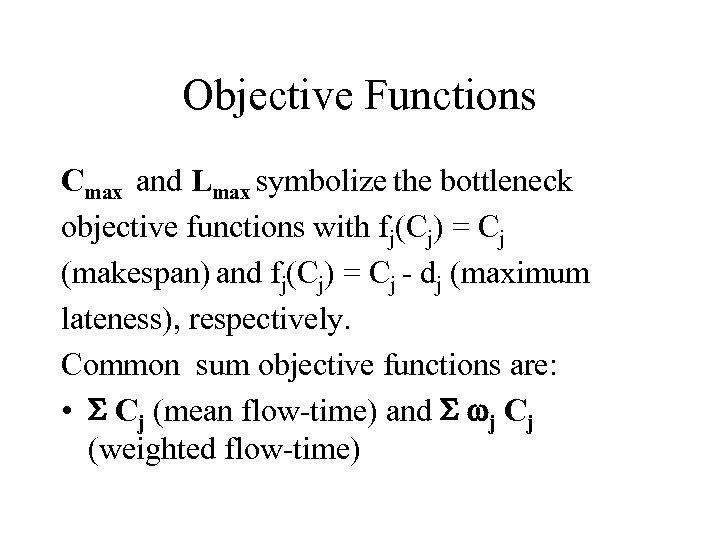Objective Functions Cmax and Lmax symbolize the bottleneck objective functions with fj(Cj) = Cj (makespan) and fj(Cj) = Cj - dj (maximum lateness), respectively. Common sum objective functions are: • S Cj (mean flow-time) and S wj Cj (weighted flow-time)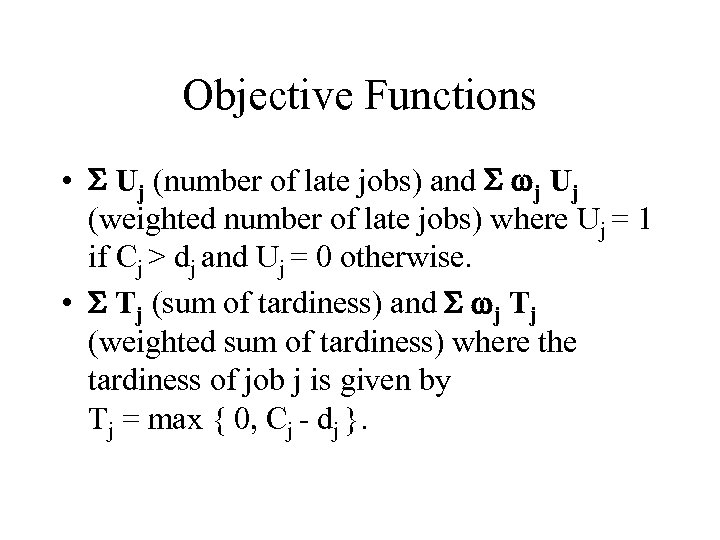Objective Functions • S Uj (number of late jobs) and S wj Uj (weighted number of late jobs) where Uj = 1 if Cj > dj and Uj = 0 otherwise. • S Tj (sum of tardiness) and S wj Tj (weighted sum of tardiness) where the tardiness of job j is given by Tj = max { 0, Cj - dj }.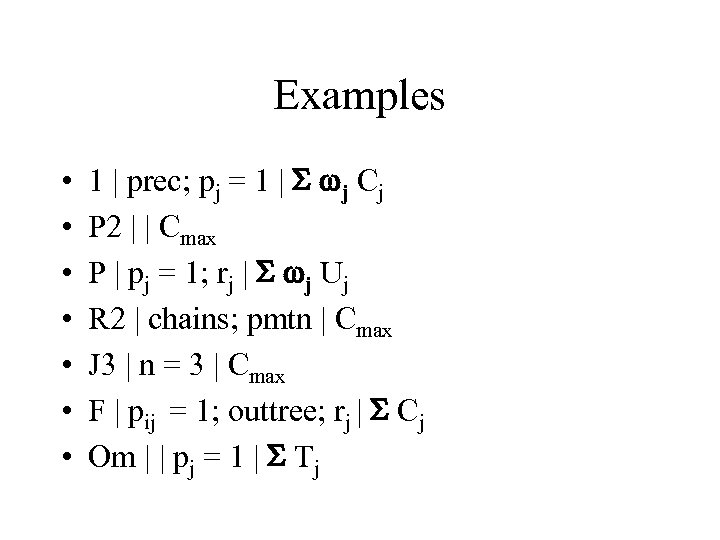Examples • • 1 | prec; pj = 1 | S wj Cj P 2 | | Cmax P | pj = 1; rj | S wj Uj R 2 | chains; pmtn | Cmax J 3 | n = 3 | Cmax F | pij = 1; outtree; rj | S Cj Om | | pj = 1 | S Tj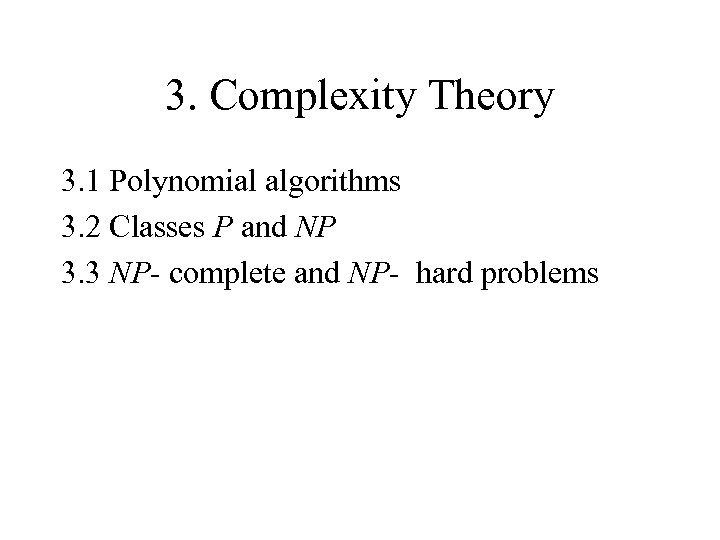3. Complexity Theory 3. 1 Polynomial algorithms 3. 2 Classes P and NP 3. 3 NP- complete and NP- hard problems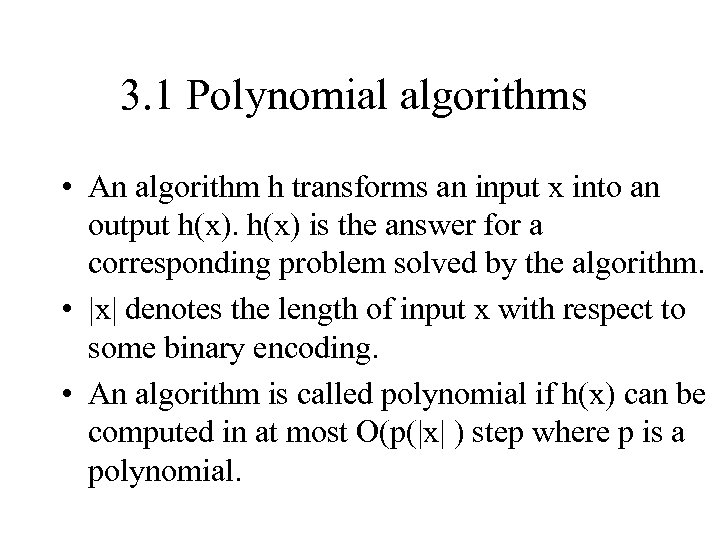3. 1 Polynomial algorithms • An algorithm h transforms an input x into an output h(x) is the answer for a corresponding problem solved by the algorithm. • |x| denotes the length of input x with respect to some binary encoding. • An algorithm is called polynomial if h(x) can be computed in at most O(p(|x| ) step where p is a polynomial.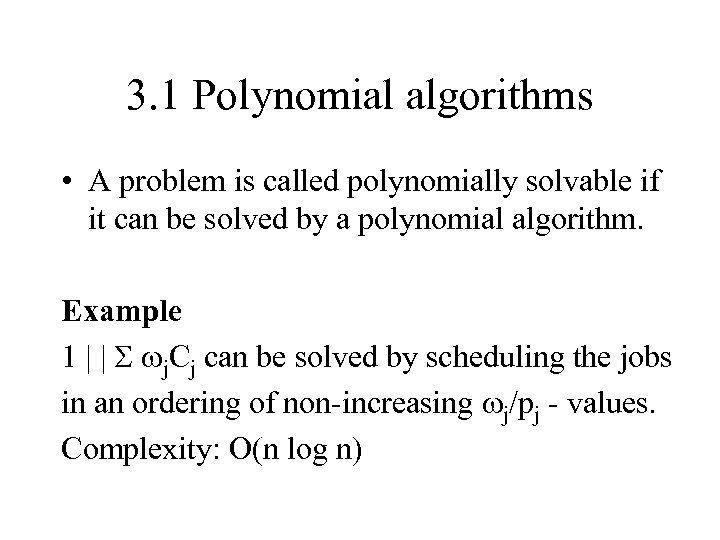3. 1 Polynomial algorithms • A problem is called polynomially solvable if it can be solved by a polynomial algorithm. Example 1 | | S wj. Cj can be solved by scheduling the jobs in an ordering of non-increasing wj/pj - values. Complexity: O(n log n)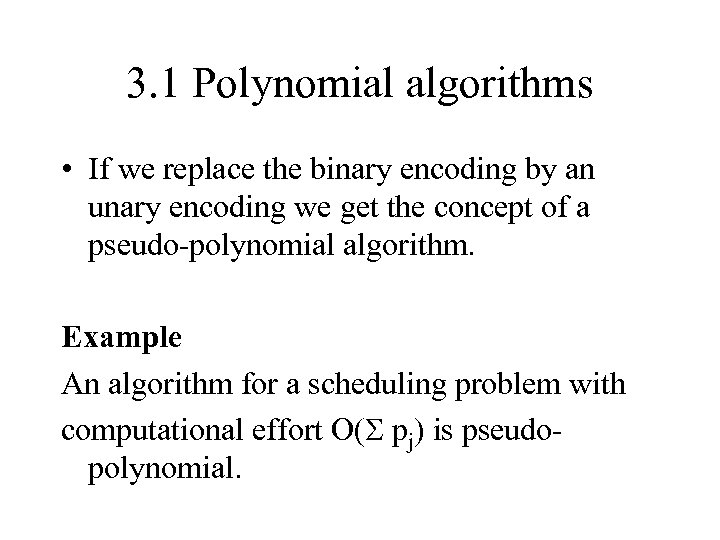3. 1 Polynomial algorithms • If we replace the binary encoding by an unary encoding we get the concept of a pseudo-polynomial algorithm. Example An algorithm for a scheduling problem with computational effort O(S pj) is pseudopolynomial.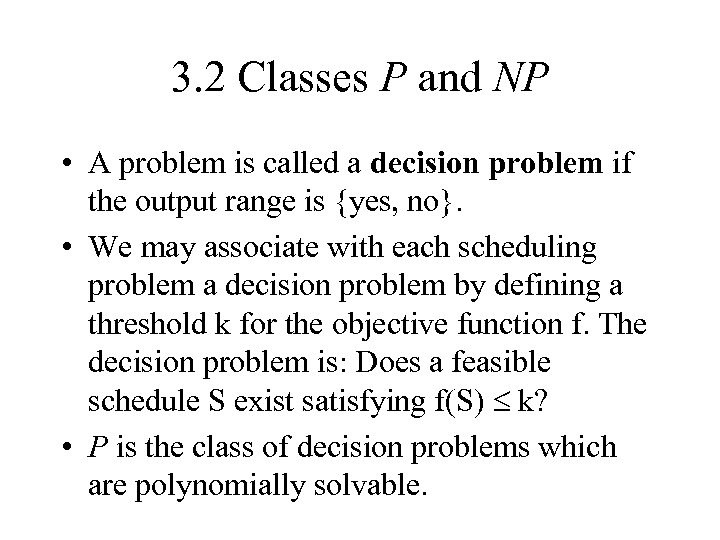3. 2 Classes P and NP • A problem is called a decision problem if the output range is {yes, no}. • We may associate with each scheduling problem a decision problem by defining a threshold k for the objective function f. The decision problem is: Does a feasible schedule S exist satisfying f(S) k? • P is the class of decision problems which are polynomially solvable.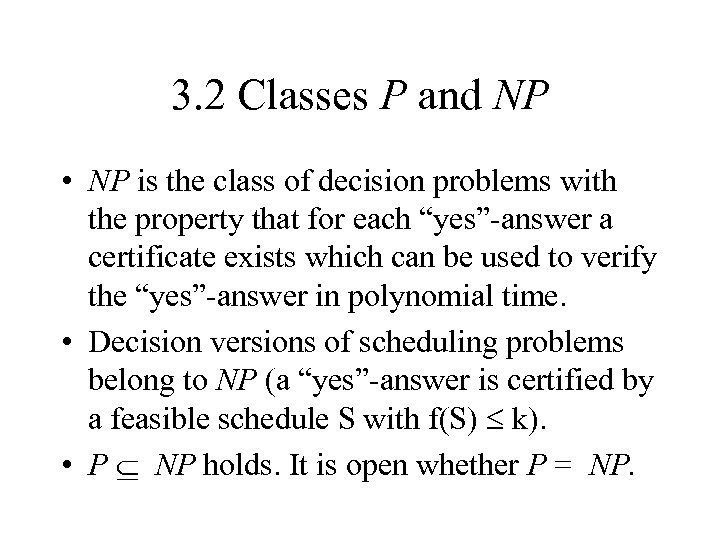3. 2 Classes P and NP • NP is the class of decision problems with the property that for each “yes”-answer a certificate exists which can be used to verify the “yes”-answer in polynomial time. • Decision versions of scheduling problems belong to NP (a “yes”-answer is certified by a feasible schedule S with f(S) k). • P NP holds. It is open whether P = NP.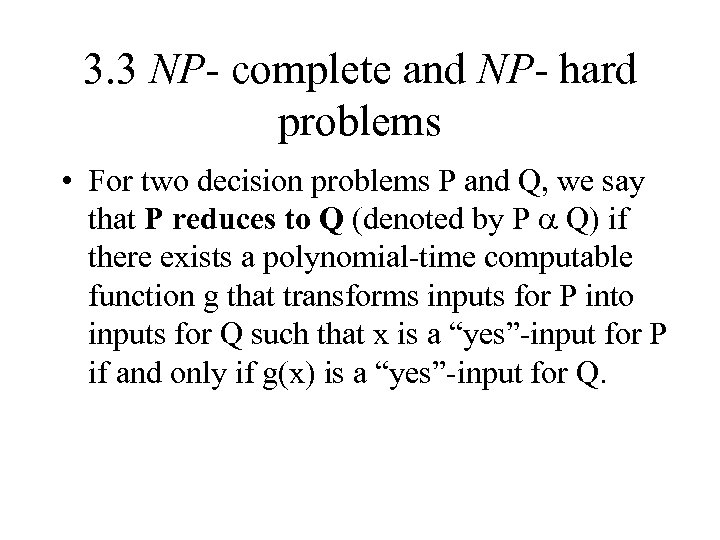3. 3 NP- complete and NP- hard problems • For two decision problems P and Q, we say that P reduces to Q (denoted by P a Q) if there exists a polynomial-time computable function g that transforms inputs for P into inputs for Q such that x is a “yes”-input for P if and only if g(x) is a “yes”-input for Q.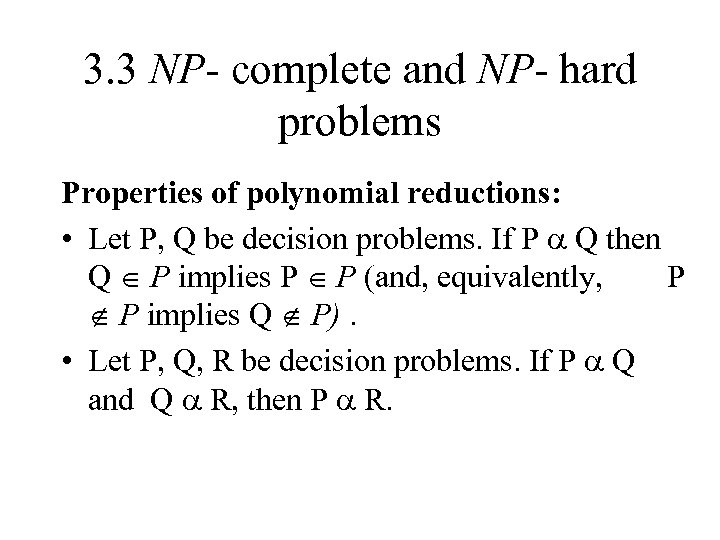3. 3 NP- complete and NP- hard problems Properties of polynomial reductions: • Let P, Q be decision problems. If P a Q then Q P implies P P (and, equivalently, P P implies Q P). • Let P, Q, R be decision problems. If P a Q and Q a R, then P a R.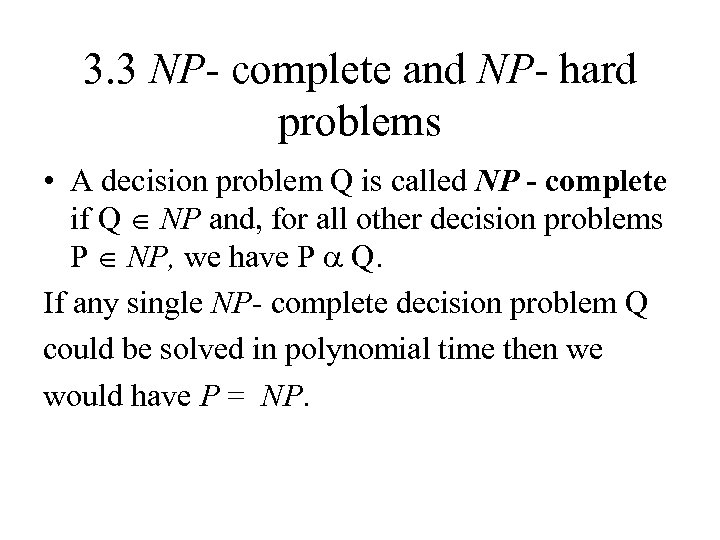3. 3 NP- complete and NP- hard problems • A decision problem Q is called NP - complete if Q NP and, for all other decision problems P NP, we have P a Q. If any single NP- complete decision problem Q could be solved in polynomial time then we would have P = NP.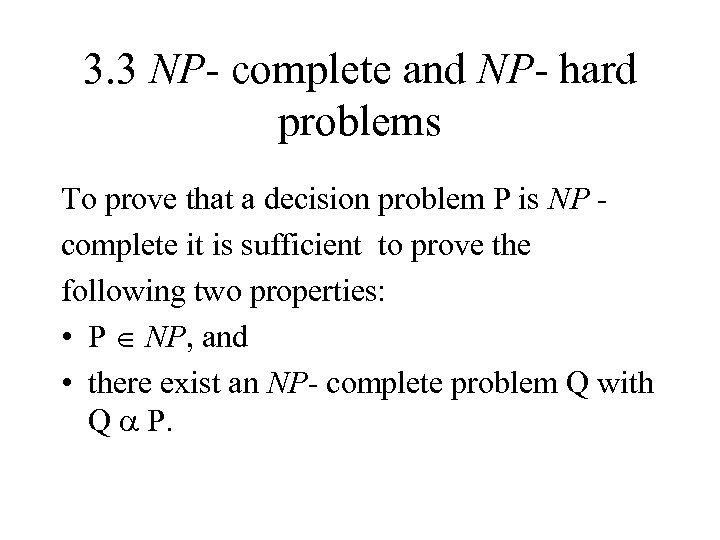3. 3 NP- complete and NP- hard problems To prove that a decision problem P is NP complete it is sufficient to prove the following two properties: • P NP, and • there exist an NP- complete problem Q with Q a P.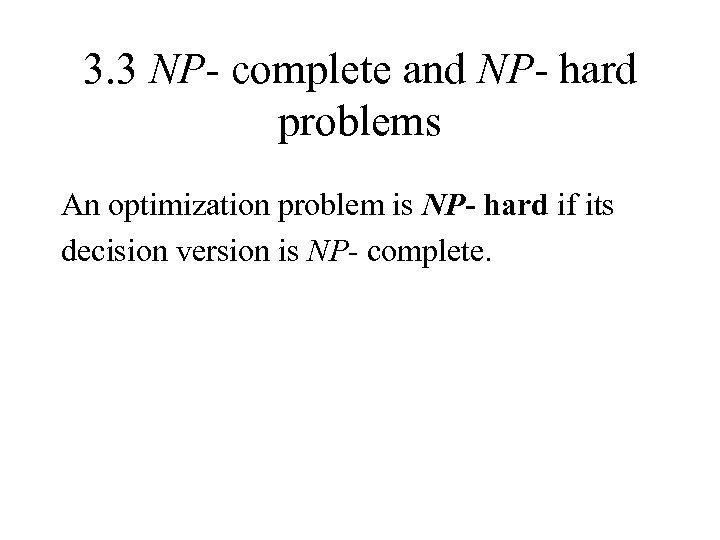3. 3 NP- complete and NP- hard problems An optimization problem is NP- hard if its decision version is NP- complete.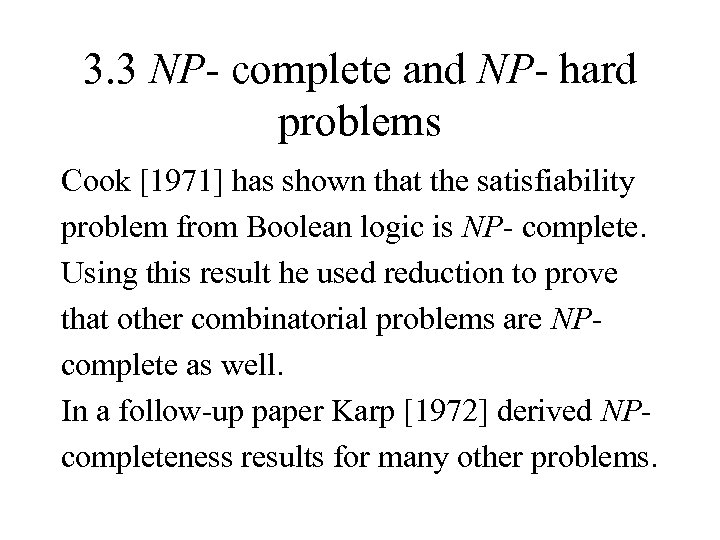3. 3 NP- complete and NP- hard problems Cook  has shown that the satisfiability problem from Boolean logic is NP- complete. Using this result he used reduction to prove that other combinatorial problems are NPcomplete as well. In a follow-up paper Karp  derived NPcompleteness results for many other problems.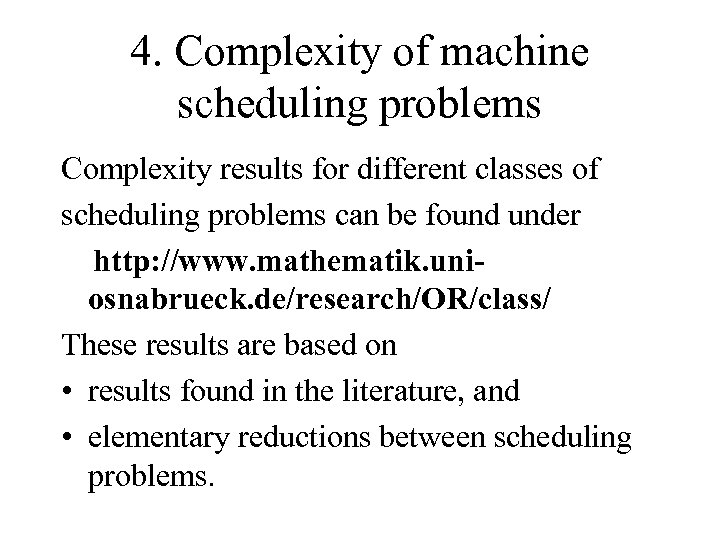4. Complexity of machine scheduling problems Complexity results for different classes of scheduling problems can be found under http: //www. mathematik. uniosnabrueck. de/research/OR/class/ These results are based on • results found in the literature, and • elementary reductions between scheduling problems.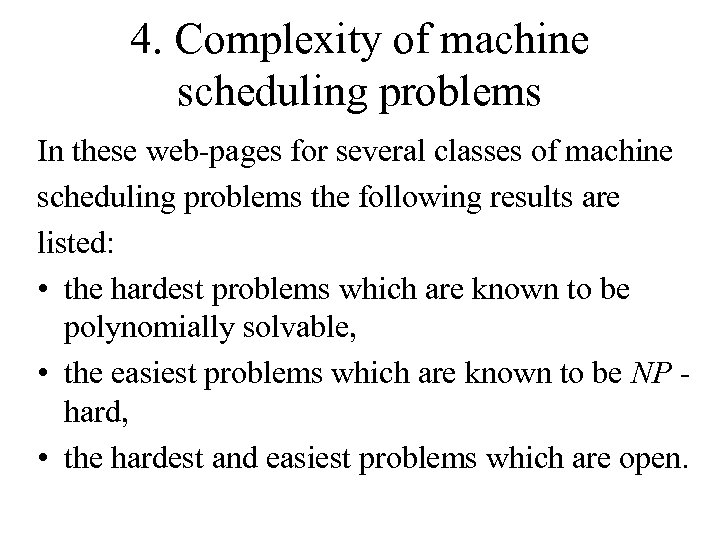4. Complexity of machine scheduling problems In these web-pages for several classes of machine scheduling problems the following results are listed: • the hardest problems which are known to be polynomially solvable, • the easiest problems which are known to be NP hard, • the hardest and easiest problems which are open.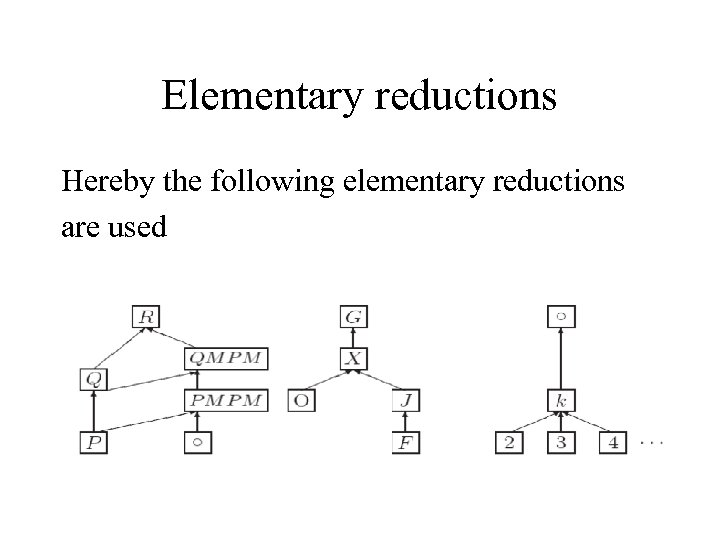Elementary reductions Hereby the following elementary reductions are used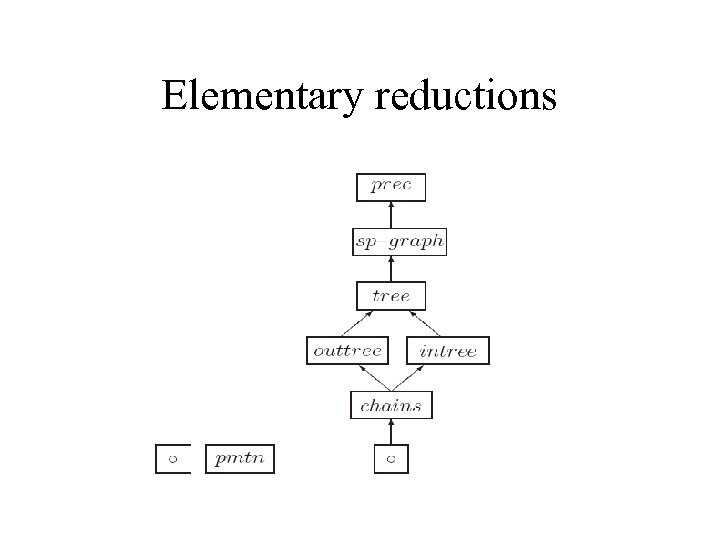Elementary reductions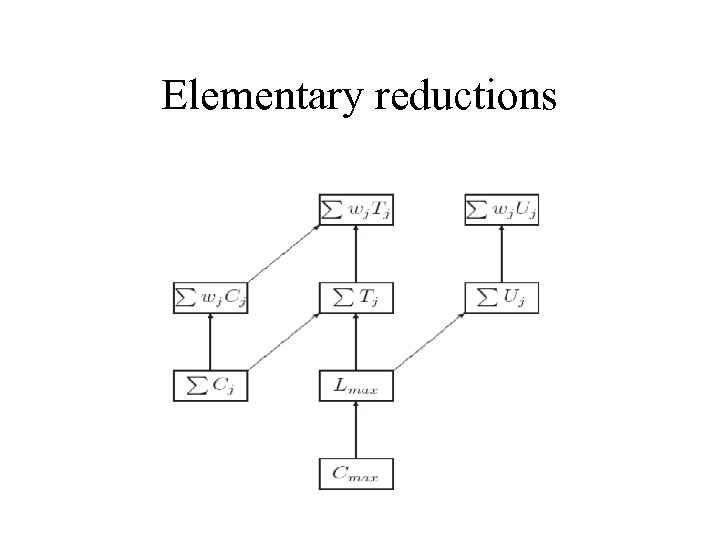Elementary reductions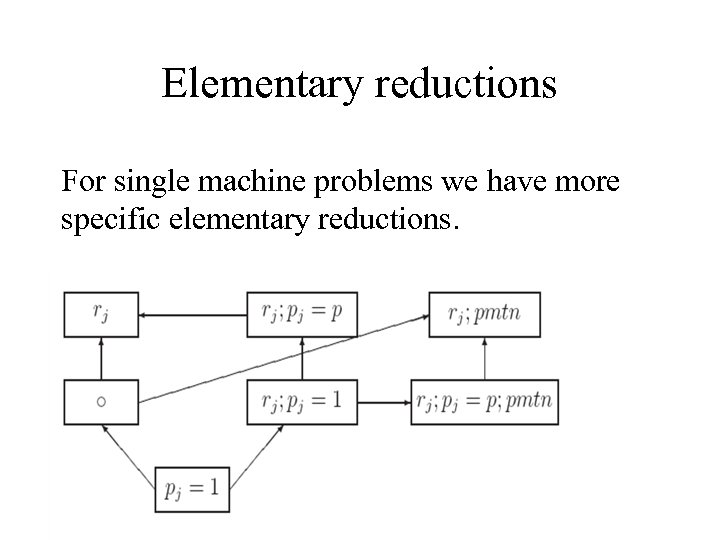Elementary reductions For single machine problems we have more specific elementary reductions.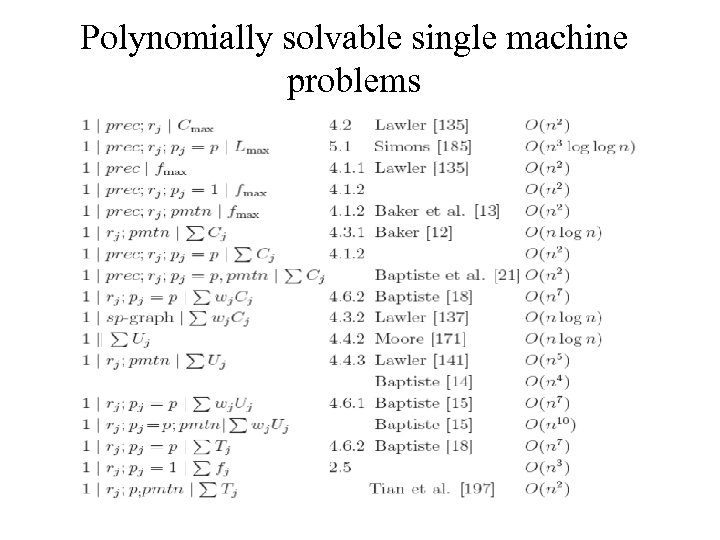Polynomially solvable single machine problems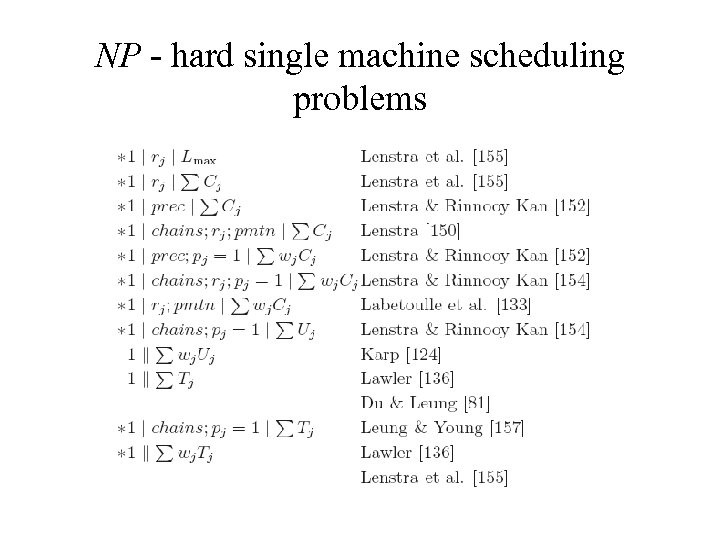NP - hard single machine scheduling problems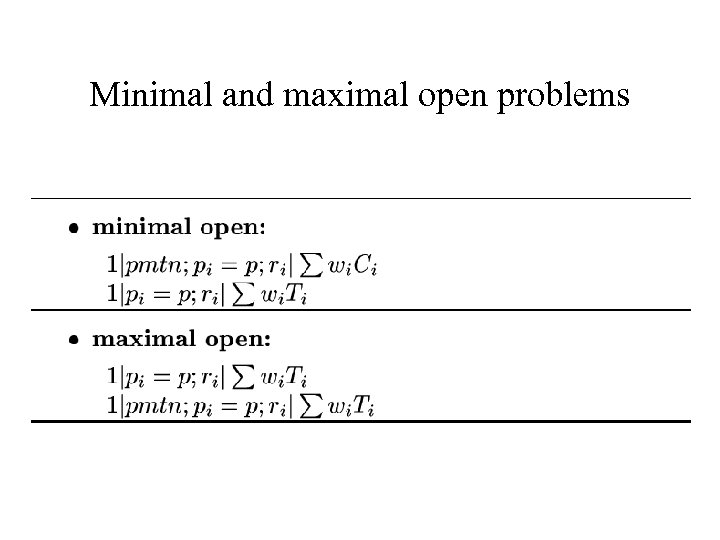Minimal and maximal open problems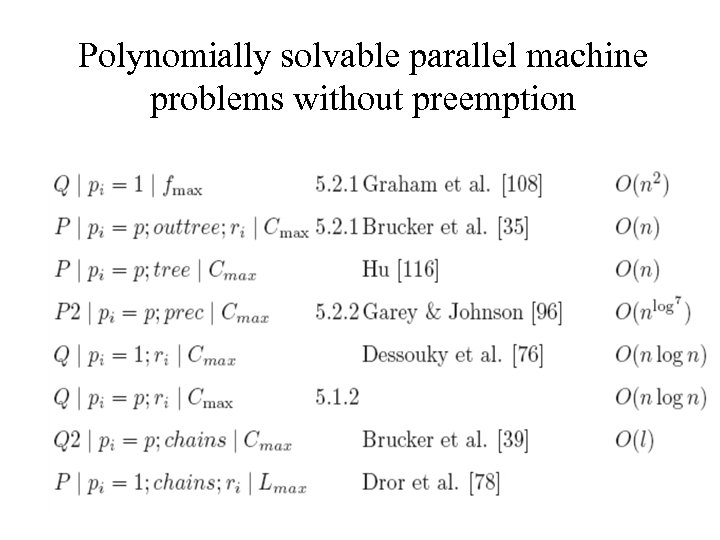Polynomially solvable parallel machine problems without preemption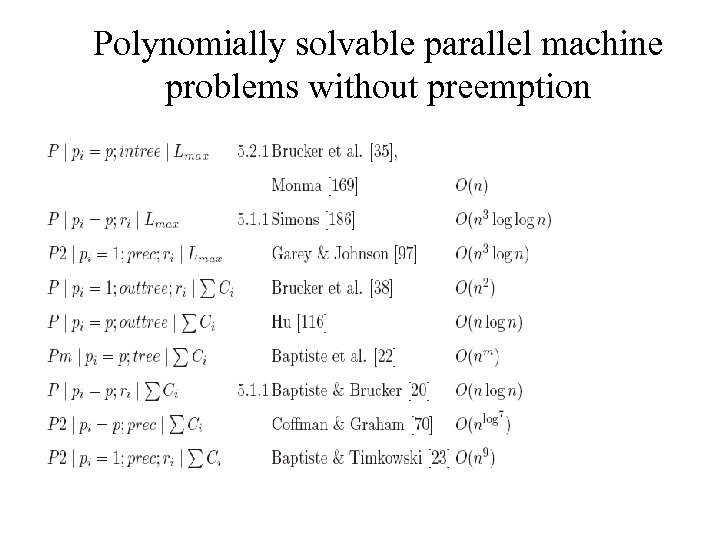Polynomially solvable parallel machine problems without preemption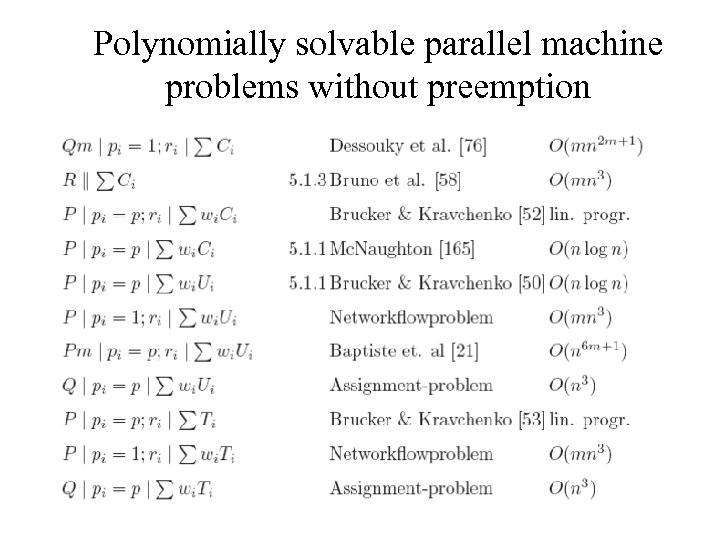Polynomially solvable parallel machine problems without preemption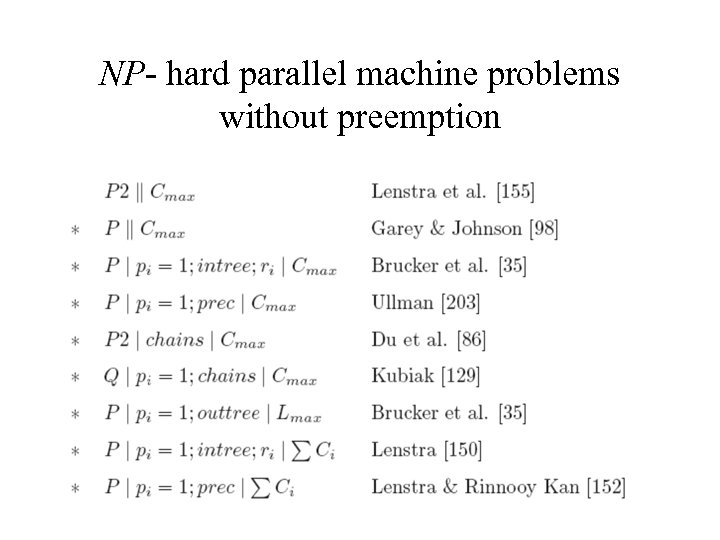NP- hard parallel machine problems without preemption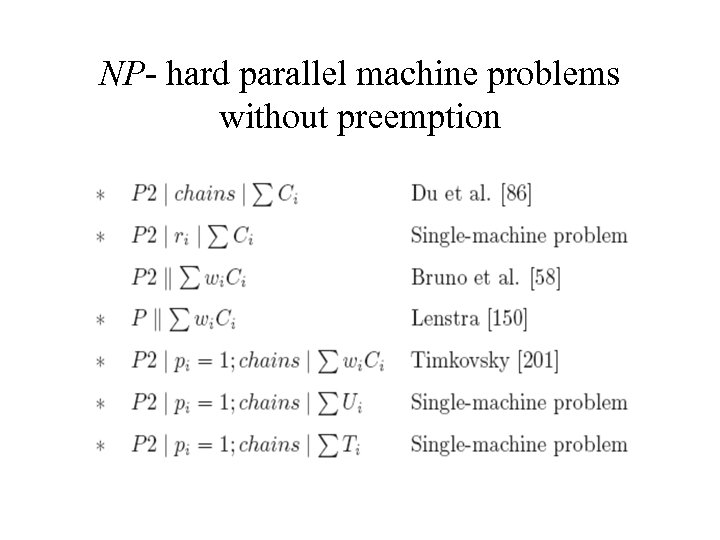NP- hard parallel machine problems without preemption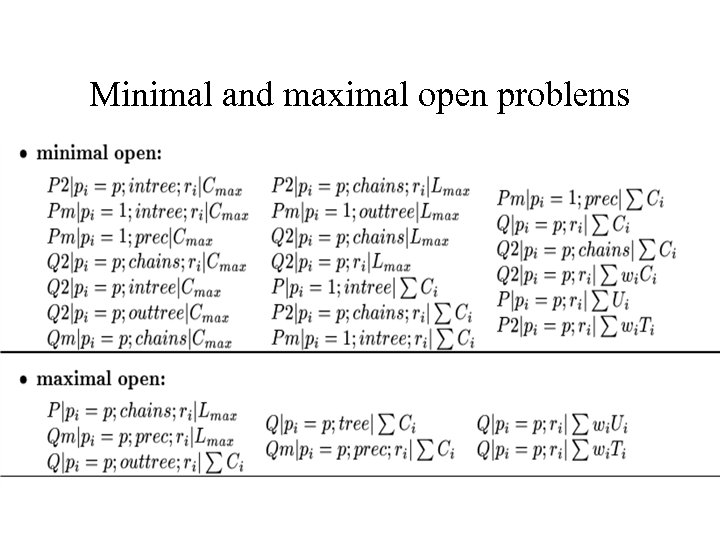Minimal and maximal open problems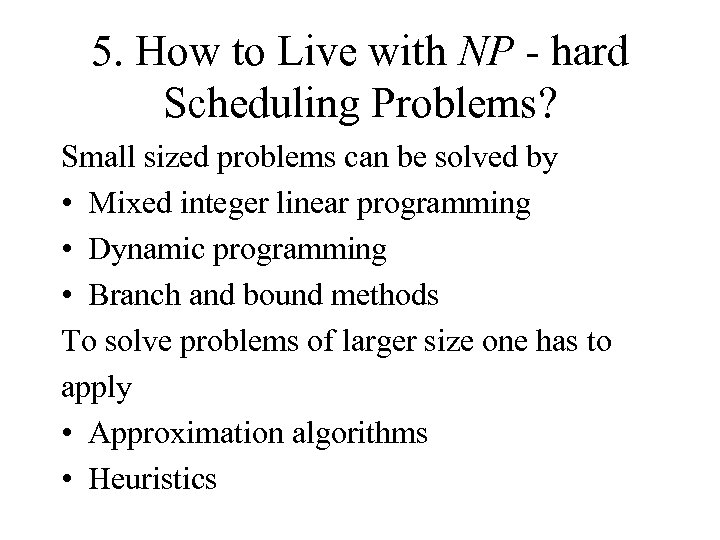5. How to Live with NP - hard Scheduling Problems? Small sized problems can be solved by • Mixed integer linear programming • Dynamic programming • Branch and bound methods To solve problems of larger size one has to apply • Approximation algorithms • Heuristics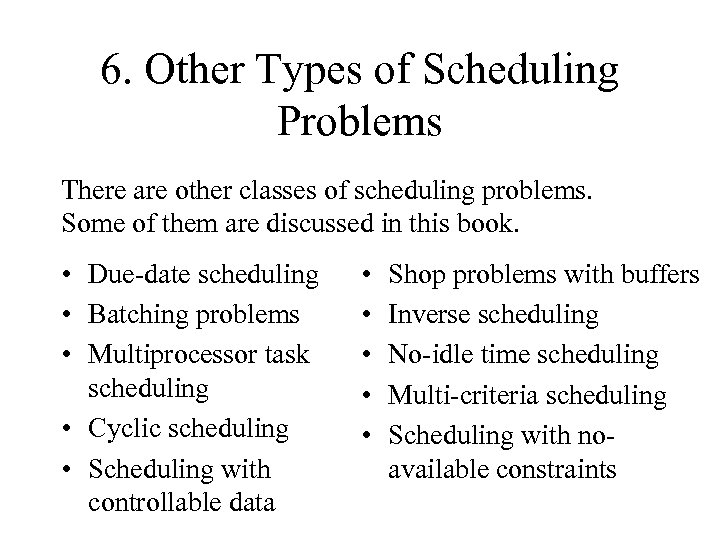6. Other Types of Scheduling Problems There are other classes of scheduling problems. Some of them are discussed in this book. • Due-date scheduling • Batching problems • Multiprocessor task scheduling • Cyclic scheduling • Scheduling with controllable data • • • Shop problems with buffers Inverse scheduling No-idle time scheduling Multi-criteria scheduling Scheduling with noavailable constraints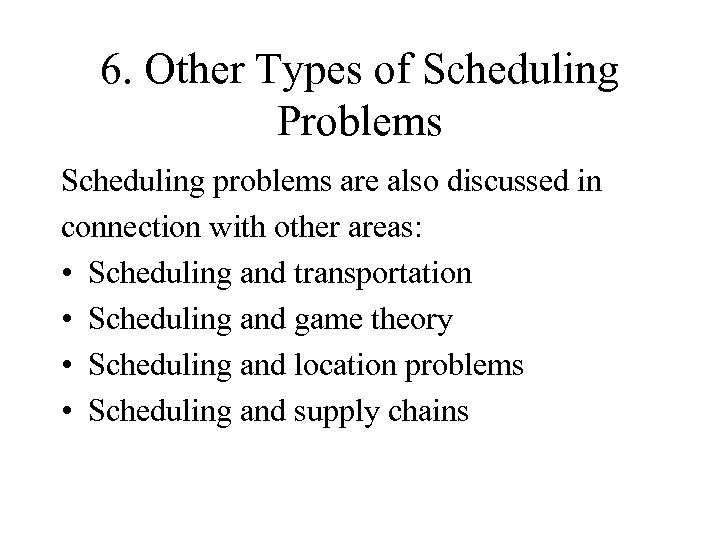6. Other Types of Scheduling Problems Scheduling problems are also discussed in connection with other areas: • Scheduling and transportation • Scheduling and game theory • Scheduling and location problems • Scheduling and supply chains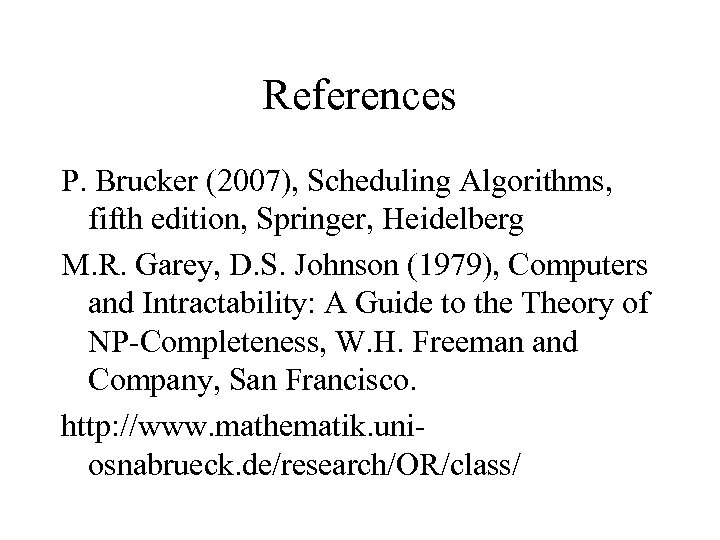References P. Brucker (2007), Scheduling Algorithms, fifth edition, Springer, Heidelberg M. R. Garey, D. S. Johnson (1979), Computers and Intractability: A Guide to the Theory of NP-Completeness, W. H. Freeman and Company, San Francisco. http: //www. mathematik. uniosnabrueck. de/research/OR/class/